# Area of a Circle Online Quiz

#### Complete Python Prime Pack

9 Courses     2 eBooks

#### Artificial Intelligence & Machine Learning Prime Pack

6 Courses     1 eBooks

#### Java Prime Pack

9 Courses     2 eBooks

Following quiz provides Multiple Choice Questions (MCQs) related to Area of a Circle. You will have to read all the given answers and click over the correct answer. If you are not sure about the answer then you can check the answer using Show Answer button. You can use Next Quiz button to check new set of questions in the quiz.Q 1 - Find the area of following circle. Use π = 3.14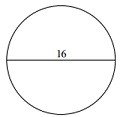### Explanation

Step 1:

Given diameter = 16 ; radius r = 16/2 = 8 units

Step 2:

Area of Circle = πr2 = (3.14)(8)(8) = 200.96 square units

Q 2 - Find the area of following circle. Use π = 3.14### Explanation

Step 1:

Given diameter = 30 ; radius r = 30/2 = 15 units

Step 2:

Area of Circle = πr2 = (3.14)(15)(15) = 706.5 square units

Q 3 - Find the area of following circle. Use π = 3.14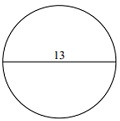### Explanation

Step 1:

Given diameter = 13 ; radius r = 13/2 = 6.5 units

Step 2:

Area of Circle = πr2 = (3.14)(6.5)(6.5) = 132.67 square units

Q 4 - Find the area of following circle. Use π = 3.14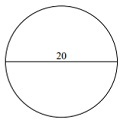### Explanation

Step 1:

Given diameter = 20 ; radius r = 20/2 = 10 units

Step 2:

Area of Circle = πr2 = (3.14)(10)(10) = 314 square units

Q 5 - Find the area of following circle. Use π = 3.14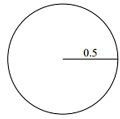### Explanation

Step 1:

Given radius r = 0.5 units

Step 2:

Area of Circle = πr2 = (3.14)(0.5)(0.5) = 0.785 square units

Q 6 - Find the area of following circle. Use π = 3.14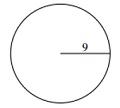### Explanation

Step 1:

Given radius r = 9 units

Step 2:

Area of Circle = πr2 = (3.14)(9)(9) = 254.34 square units

Q 7 - Find the area of following circle. Use π = 3.14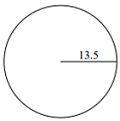### Explanation

Step 1:

Given radius r = 13.5 units

Step 2:

Area of Circle = πr2 = (3.14)(13.5)(13.5) = 572.27 square units

Q 8 - Find the area of following circle. Use π = 3.14### Explanation

Step 1:

Given radius r = 6 units

Step 2:

Area of Circle = πr2 = (3.14)(6)(6) = 113.04 square units

Q 9 - Find the area of following circle. Use π = 3.14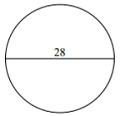### Explanation

Step 1:

Given diameter = 28 ; radius r = 28/2 = 14 units

Step 2:

Area of Circle = πr2 = (3.14)(14)(14) = 615.44 square units

Q 10 - Find the area of following circle. Use π = 3.14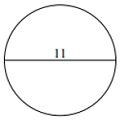### Explanation

Step 1:

Given diameter = 11 ; radius r = 11/2 = 5.5 units

Step 2:

Area of Circle = πr2 = (3.14)(5.5)(5.5) = 94.99 square units

area_of_circle.htm